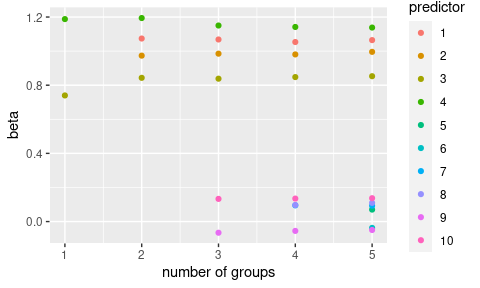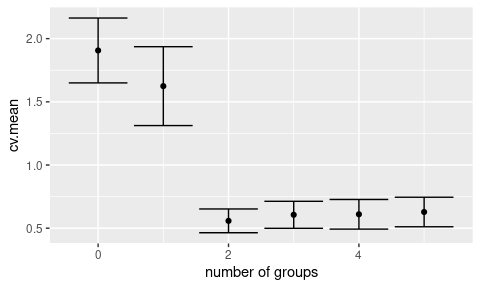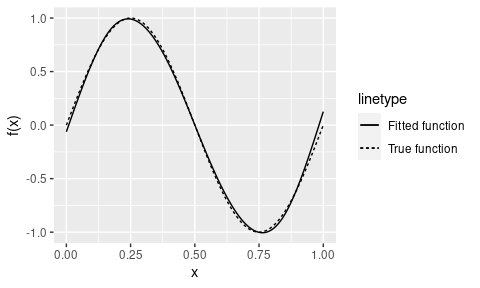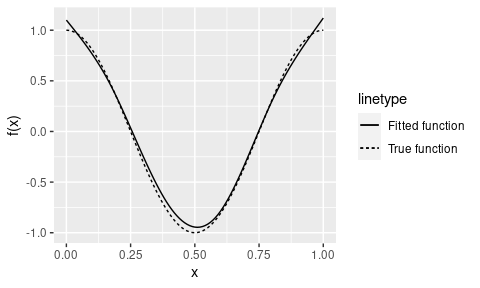# Guide to grpsel

## Introduction

grpsel is an R package for group subset selection (see https://arxiv.org/abs/2105.12081). For a response vector $$\mathbf{y}=(y_1,\ldots,y_n)^\top$$, predictor matrix $$\mathbf{X}=(\mathbf{x}_1,\ldots,\mathbf{x}_n)^\top$$, and a set of $$g$$ groups, grpsel is capable of approximately solving problems of the form: $\min_\beta \sum_{i=1}^n\ell(\mathbf{x}_i^\top\beta,y_i)+\lambda\sum_{k=1}^g1(\|\beta_k\|\neq0)+\gamma\sum_{k=1}^g\|\beta_k\|^q,\quad q\in\{1,2\}$ where the first term is a loss function (square or logistic), the second term is a group subset selection penalty, and the third term is a shrinkage penalty (specifically, a group lasso penalty if $$q=1$$ and a ridge penalty if $$q=2$$). The notation $$\beta_k$$ denotes the coefficients $$\beta$$ belonging to the $$k$$th group.

Group-sparse regression arises in numerous settings in modern data analytic work, including selection with categorical predictors, multitask (multiresponse) learning, hierarchical selection, and nonparametric additive regression. We demonstrate some examples of these applications below.

The grpsel package provides a simple set of functions for handling grouped selection in R. The two main functions provided by the package are grpsel() and cv.grpsel(), responsible for model fitting and cross-validation, respectively.

The grpsel() function provides a convenient way of performing group subset selection for a path of $$\lambda$$. To demonstrate this functionality, let’s simulate some grouped data.

set.seed(123)
n <- 100 # Number of observations
p <- 10 # Number of predictors
g <- 5 # Number of groups
group <- rep(1:g, each = p / g) # Group structure
beta <- numeric(p)
beta[which(group %in% 1:2)] <- 1 # First two groups are nonzero
x <- matrix(rnorm(n * p), n, p)
y <- x %*% beta + rnorm(n)

The first two groups explain the response while the rest are unimportant.

library(grpsel)
fit <- grpsel(x, y, group)

The group argument is optional, and if left unspecified, each predictor will be assigned to its own group (leading to ungrouped variable selection).

The values of $$\lambda$$ are automatically computed from the data, providing a path of solutions from the null model to the full model. These solutions can be extracted using the coef() function.

coef(fit)
#>            [,1]      [,2]      [,3]        [,4]        [,5]        [,6]
#>  [1,] 0.1870419 0.1409381 0.1363219  0.14577657  0.14818803  0.13693870
#>  [2,] 0.0000000 0.0000000 1.0738565  1.06824820  1.05330570  1.06458112
#>  [3,] 0.0000000 0.0000000 0.9734311  0.98498345  0.98057908  0.99567892
#>  [4,] 0.0000000 0.7399178 0.8432186  0.83820508  0.84769346  0.85285167
#>  [5,] 0.0000000 1.1879367 1.1940502  1.15017753  1.14165312  1.13815099
#>  [6,] 0.0000000 0.0000000 0.0000000  0.00000000  0.00000000  0.07012182
#>  [7,] 0.0000000 0.0000000 0.0000000  0.00000000  0.00000000 -0.03724315
#>  [8,] 0.0000000 0.0000000 0.0000000  0.00000000  0.09499731  0.09296110
#>  [9,] 0.0000000 0.0000000 0.0000000  0.00000000  0.09697281  0.10961805
#> [10,] 0.0000000 0.0000000 0.0000000 -0.06559522 -0.05491667 -0.04951346
#> [11,] 0.0000000 0.0000000 0.0000000  0.13293905  0.13484139  0.13699716

Each of the columns above corresponds to a set of estimated coefficients for a particular value of $$\lambda$$, with the first row containing the intercept terms. These coefficients can be visualised via the plot() function.

plot(fit)The plot above omits the intercept terms and displays the coefficients by the number of selected groups rather than $$\lambda$$.

The predict() function is available to make predictions for new data.

x.new <- matrix(rnorm(10 * p), 10, p)
predict(fit, x.new)
#>            [,1]       [,2]       [,3]       [,4]       [,5]       [,6]
#>  [1,] 0.1870419  0.2474754  0.5547334  0.4828247  0.5505698  0.5159474
#>  [2,] 0.1870419 -1.0364437  0.6014120  0.9496678  1.0179290  1.0539641
#>  [3,] 0.1870419  1.7299335  3.2266509  3.5362877  3.4558829  3.4516054
#>  [4,] 0.1870419 -1.1671608 -3.5933701 -3.4910744 -3.4566040 -3.4945212
#>  [5,] 0.1870419 -1.4625154 -0.3259065 -0.4122397 -0.4797968 -0.5027092
#>  [6,] 0.1870419  1.6731365  2.5022256  2.4825564  2.4170965  2.3978358
#>  [7,] 0.1870419 -0.8084083 -1.9495455 -1.9342569 -1.9434672 -2.0253390
#>  [8,] 0.1870419  1.3557892  0.9618111  1.1240626  1.1827934  1.2321732
#>  [9,] 0.1870419  2.2387387  2.3907195  2.1533365  1.8768519  1.8623801
#> [10,] 0.1870419 -1.2059448 -3.1525808 -2.9586382 -2.9207032 -2.9395051

Again, the columns represent predictions for different values of $$\lambda$$.

By default, grpsel sets $$\gamma=0$$. In certain situations, the shrinkage induced by the setting $$\gamma>0$$ is desirable (e.g., when the level of noise is high). To fit the model for both $$\lambda$$ and $$\gamma$$, use the argument penalty='grSubset+grLasso' or penalty='grSubset+Ridge'. To extract the coefficients for specific values of $$\lambda$$ and $$\gamma$$, the lambda and gamma arguments of coef() are available.

fit <- grpsel(x, y, group, penalty = 'grSubset+grLasso')
coef(fit, lambda = 0.05, gamma = 0.1)
#>            [,1]
#>  [1,] 0.1474263
#>  [2,] 0.9059429
#>  [3,] 0.8479202
#>  [4,] 0.7198753
#>  [5,] 1.0439736
#>  [6,] 0.0000000
#>  [7,] 0.0000000
#>  [8,] 0.0000000
#>  [9,] 0.0000000
#> [10,] 0.0000000
#> [11,] 0.0000000

fit <- grpsel(x, y, group, penalty = 'grSubset+Ridge')
coef(fit, lambda = 0.05, gamma = 0.1)
#>            [,1]
#>  [1,] 0.1442755
#>  [2,] 0.9632668
#>  [3,] 0.8929407
#>  [4,] 0.7565775
#>  [5,] 1.0884522
#>  [6,] 0.0000000
#>  [7,] 0.0000000
#>  [8,] 0.0000000
#>  [9,] 0.0000000
#> [10,] 0.0000000
#> [11,] 0.0000000

Similar arguments exist for predict().

In practice, $$\lambda$$ and $$\gamma$$ usually need to be cross-validated. The cv.grpsel() function provides a convenient way to perform group subset selection with cross-validation.

fit <- cv.grpsel(x, y, group, penalty = 'grSubset+Ridge', nfold = 10) # 10-fold cross-validation

The cross-validation results are easily visualised using the plot() function.

plot(fit)The plot above shows the cross-validated loss (square or logistic) as a function of the number of selected groups for the best cross-validated value of $$\gamma$$. Plots for other values of $$\gamma$$ can be produced using the gamma argument of plot().

The coef() and predict() functions applied to the output of cv.grpsel() return results corresponding to the values of $$\lambda$$ and $$\gamma$$ that minimise the cross-validated mean square error.

coef(fit)
#>            [,1]
#>  [1,] 0.1363415
#>  [2,] 1.0735914
#>  [3,] 0.9732420
#>  [4,] 0.8430108
#>  [5,] 1.1938004
#>  [6,] 0.0000000
#>  [7,] 0.0000000
#>  [8,] 0.0000000
#>  [9,] 0.0000000
#> [10,] 0.0000000
#> [11,] 0.0000000
predict(fit, x.new)
#>             [,1]
#>  [1,]  0.5546395
#>  [2,]  0.6013611
#>  [3,]  3.2260534
#>  [4,] -3.5925209
#>  [5,] -0.3257647
#>  [6,]  2.5016732
#>  [7,] -1.9490490
#>  [8,]  0.9616619
#>  [9,]  2.3903344
#> [10,] -3.1518596

grpsel() does not need to be run after using cv.grpsel(), as the latter calls the former and saves the result as fit\$fit.

Finally, to perform a logistic regression fit, set loss='logistic'.

y <- rbinom(n, 1, 1 / (1 + exp(- x %*% beta)))
fit <- cv.grpsel(x, y, group, loss = 'logistic')
coef(fit)
#>            [,1]
#>  [1,] 0.2634796
#>  [2,] 1.1012157
#>  [3,] 1.0824249
#>  [4,] 1.3042438
#>  [5,] 0.8973998
#>  [6,] 0.0000000
#>  [7,] 0.0000000
#>  [8,] 0.0000000
#>  [9,] 0.0000000
#> [10,] 0.0000000
#> [11,] 0.0000000

## Overlapping groups

It is straightforward to model overlapping groups using grpsel. To demonstrate, suppose there are ten predictors spread among two groups: $$\{1,2,3,4,5,6\}$$ and $$\{5,6,7,8,9,10\}$$, where $$x_5$$ and $$x_6$$ belong to both groups.

x <- matrix(rnorm(n * p), n, p)
y <- rowSums(x) + rnorm(n)
group <- list(1:6, 5:10)
fit <- grpsel(x, y, group)

The object group is now a list of groups rather than a vector.

coef(fit)
#>             [,1]       [,2]        [,3]
#>  [1,] 0.02193046 -0.3513713 -0.05257854
#>  [2,] 0.00000000  1.0590892  0.96774167
#>  [3,] 0.00000000  1.1276923  1.15569494
#>  [4,] 0.00000000  0.8306162  0.96424793
#>  [5,] 0.00000000  1.0351453  1.11730453
#>  [6,] 0.00000000  1.1722285  1.11375229
#>  [7,] 0.00000000  0.8029290  0.96864748
#>  [8,] 0.00000000  0.0000000  0.88225886
#>  [9,] 0.00000000  0.0000000  1.04247927
#> [10,] 0.00000000  0.0000000  0.87981630
#> [11,] 0.00000000  0.0000000  0.92065396

Under the hood, the overlapping groups are handled using a latent coefficient approach. See https://arxiv.org/abs/2105.12081 for more information.

## Algorithms

The primary algorithm driving grpsel is coordinate descent. Sometimes when the groups are strongly correlated, the estimate produced by coordinate descent can be improved using local search. This algorithm runs on top of coordinate descent. To use local search, set local.search=T.

group <- rep(1:g, each = p / g)
x <- matrix(rnorm(n * p), n, p) + matrix(rnorm(n), n, p)
beta[which(group %in% 1:2)] <- 1 # First two groups are nonzero
y <- x %*% beta + rnorm(n)
fit <- cv.grpsel(x, y, group)
coef(fit)
#>              [,1]
#>  [1,] -0.01635903
#>  [2,]  0.95557006
#>  [3,]  1.24236451
#>  [4,]  0.92888981
#>  [5,]  0.78221128
#>  [6,]  0.13708882
#>  [7,]  0.18160260
#>  [8,] -0.21054320
#>  [9,] -0.03611143
#> [10,]  0.00000000
#> [11,]  0.00000000
fit <- cv.grpsel(x, y, group, local.search = T)
coef(fit)
#>             [,1]
#>  [1,] 0.02690425
#>  [2,] 0.98993334
#>  [3,] 1.20206912
#>  [4,] 0.98561726
#>  [5,] 0.81895840
#>  [6,] 0.00000000
#>  [7,] 0.00000000
#>  [8,] 0.00000000
#>  [9,] 0.00000000
#> [10,] 0.00000000
#> [11,] 0.00000000

The correct groups are not selected without local search in this high-correlation example.

## Demonstrations

In this section, we show how grpsel can be used to fit a variety of statistical models.

Multitask learning is useful where the response is a matrix and it is suspected that each of its columns can be explained by the same subset of predictors. In this case, we would like to perform a single fit rather than individual fits on each column.

In this example, we will simulate ten response variables, each depending on the first five predictors.

m <- 10 # Number of response variables
beta <- matrix(0, p, m)
beta[1:5, ] <- 1
x <- matrix(rnorm(n * p), n, p)
y <- x %*% beta + matrix(rnorm(n * m), n, m)

y <- matrix(y, ncol = 1)
x <- diag(m) %x% x
group <- rep(1:p, m)

cvfit <- cv.grpsel(x, y, group)
matrix(coef(cvfit)[- 1, , drop = F], ncol = m)
#>            [,1]      [,2]      [,3]      [,4]      [,5]      [,6]      [,7]
#>  [1,] 1.0338013 0.8942857 0.8882466 0.9107143 1.0034279 1.1095664 1.0604750
#>  [2,] 1.1239842 0.8762186 0.9103406 1.0858450 0.9095743 1.0419114 1.2156836
#>  [3,] 0.9980841 1.0808339 0.9224392 0.9451404 1.1005818 1.0579970 0.9697167
#>  [4,] 0.8153082 0.9034146 1.0668537 0.8761911 1.0157048 1.0683427 0.9269141
#>  [5,] 0.8864655 0.7751244 0.9415790 1.0631036 0.9908681 0.8868114 0.9441526
#>  [6,] 0.0000000 0.0000000 0.0000000 0.0000000 0.0000000 0.0000000 0.0000000
#>  [7,] 0.0000000 0.0000000 0.0000000 0.0000000 0.0000000 0.0000000 0.0000000
#>  [8,] 0.0000000 0.0000000 0.0000000 0.0000000 0.0000000 0.0000000 0.0000000
#>  [9,] 0.0000000 0.0000000 0.0000000 0.0000000 0.0000000 0.0000000 0.0000000
#> [10,] 0.0000000 0.0000000 0.0000000 0.0000000 0.0000000 0.0000000 0.0000000
#>            [,8]      [,9]     [,10]
#>  [1,] 1.0611635 1.3355557 0.9833819
#>  [2,] 1.0456646 1.0476437 1.0841175
#>  [3,] 0.9171092 0.7631579 0.9177739
#>  [4,] 1.0303281 0.9229071 0.7567556
#>  [5,] 0.8862811 1.1295866 1.0961600
#>  [6,] 0.0000000 0.0000000 0.0000000
#>  [7,] 0.0000000 0.0000000 0.0000000
#>  [8,] 0.0000000 0.0000000 0.0000000
#>  [9,] 0.0000000 0.0000000 0.0000000
#> [10,] 0.0000000 0.0000000 0.0000000

The groups have enforced the constraint that all ten response variables share the same subset of predictors.

Group selection can be used to fit sparse nonparametric additive models of the form $y=\sum_{j=1}^pf_j(x_j)+\epsilon.$ To fit these models we can approximate $$f_1,\ldots,f_p$$ using regression splines. In this example, the predictors are uniform on $$[0,1]$$ and the generating functions are \begin{aligned} f_1(x) &= \sin(2\pi x), \\ f_2(x) &= \cos(2\pi x), \\ f_3(x) &= 0,\,\text{and} \\ &~~\vdots \\ f_p(x) &= 0. \end{aligned} We will use natural cubic splines with five basis functions to fit this model. The basis functions of each spline form a group, and we have one such group for each predictor.

x <- matrix(runif(n * p), n, p)
y <- sinpi(2 * x[, 1]) + cospi(2 * x[, 2]) + rnorm(n, sd = 0.1)
df <- 5
splines <- lapply(1:p, $$j) splines::ns(x[, j], df = df)) x.s <- do.call(cbind, splines) group <- rep(1:p, each = df) fit <- cv.grpsel(x.s, y, group) Let’s check that the first two predictors have been selected correctly. unique(group[coef(fit)[- 1] != 0]) #>  1 2 The fitted functions can be plotted as follows. library(ggplot2) beta0 <- coef(fit) beta <- coef(fit)[- 1] int.x1 <- (beta0 + colMeans(x.s[, - (1:df)]) %*% beta[- (1:df)])[, ] int.x2 <- (beta0 + colMeans(x.s[, - (1:df + df)]) %*% beta[- (1:df + df)])[, ] ggplot(x = seq(- 1, 1, length.out = 101)) + stat_function(fun = \(x) sinpi(2 * x), aes(linetype = 'True function')) + stat_function(fun = \(x) int.x1 + predict(splines[], x) %*% beta[1:df], aes(linetype = 'Fitted function')) + xlab('x') + ylab('f(x)')ggplot(x = seq(- 1, 1, length.out = 101)) + stat_function(fun = \(x) cospi(2 * x), aes(linetype = 'True function')) + stat_function(fun = \(x) int.x2 + predict(splines[], x) %*% beta[1:df + df], aes(linetype = 'Fitted function')) + xlab('x') + ylab('f(x)')### Hierarchical interactions When modelling interactions between predictors, it is often desirable to enforce the condition that an interaction can be selected only when its constituent predictors are selected, i.e., the coefficient on \(x_1x_2$$ can be nonzero only when the coefficients on $$x_1$$ and $$x_2$$ are nonzero. It is straightforward to enforce this hierarchical constraint using group selection.

In this example, the main effects $$x_1$$, $$x_2$$, and $$x_3$$ are nonzero, as well as the interaction $$x_1x_2$$.

x <- matrix(rnorm(n * p), n, p)
y <- x[, 1] + x[, 2] + x[, 3] + x[, 1] * x[, 2] + rnorm(n, sd = 0.1)

x.int <- model.matrix(~ - 1 + . ^ 2, data = as.data.frame(x))
group <- c(1:p, mapply(c, combn(1:p, 2, simplify = F), 1:choose(p, 2) + p, SIMPLIFY = F))
fit <- cv.grpsel(x.int, y, group)
colnames(x.int)[coef(fit)[- 1] != 0]
#>  "V1"    "V2"    "V3"    "V1:V2"

The fitted model respects the hierarchy constraint.